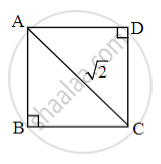# Choose the correct alternative: If the length of diagonal of square is √2, then what is the length of each side? - Geometry

MCQ

Choose the correct alternative:

If the length of diagonal of square is √2, then what is the length of each side?

• 2

• √3

• 1

• 4

#### Solution

1Let ABCD be the given square.

In ∆ABC,

∠B = 90°

∴ AC2 = AB2 + BC2     ...[Pythagoras theorem]

∴ (√2)2 = AB2 + AB2     ...[Sides of the square]

∴ 2 = 2AB2

∴ AB2 = 1

∴ AB = 1 unit

Concept: Converse of Pythagoras Theorem
Is there an error in this question or solution?
Chapter 2: Pythagoras Theorem - Q.1 (A)

Share